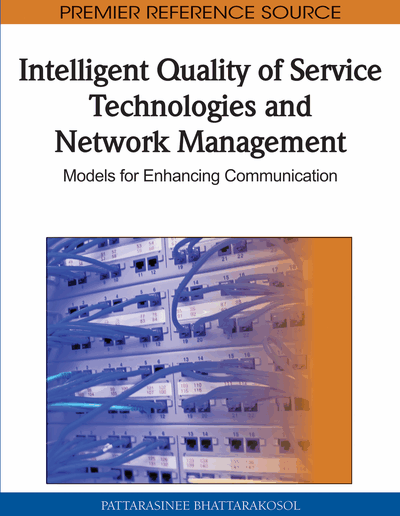# Multiple Optimization of Network Carrier and Traffic Flow Goals Using a Heuristic Routing Decision System

Wayne S. Goodridge (University of West Indies, Trinidad and Tobago), Shyamala C. Sivakumar (Saint Mary’s University, Canada), William Robertson (Dalhousie University, Canada) and William J. Phillips (Dalhousie University, Canada)
DOI: 10.4018/978-1-61520-791-6.ch007
Available
\$37.50
No Current Special Offers

## Abstract

This chapter presents a multiple constraint optimization algorithm called routing decision system (RDS) that uses the concept of preference functions to address the problem of selecting paths in core networks that satisfy traffic-oriented QoS requirements while simultaneously satisfying network resource-oriented performance goals. The original contribution lies in the use of strong scales employed for constructing a multiple criteria preference function in an affine space. The use of preference functions makes it possible for paths that match both traffic-oriented and resource-oriented goals to be selected by the algorithm. The RDS algorithm is used in conjunction with a heuristic path finding algorithm called Constraint Path Heuristic (CP-H) algorithm which is a novel approach to finding a set of constraint paths between source and destination nodes in a network. The CP-H algorithm finds multiple paths for each metric and then passes all the paths to the RDS algorithm. Simulation results showed that the CP-H/RDS algorithm has a success rate of between 93 and 96% when used in Waxman graph topologies, and is shown to be significantly better than other heuristic based algorithms under strict constraints. In addition, it is shown that the associated execution time of the CP-H/RDS algorithm is slightly higher than other heuristic based algorithms but good enough for use in an online traffic engineering (TE) application. Simulations to assess the performance of CP-H/RDS algorithm in a TE environment show that the algorithms has lower call block rates than other TE algorithms. It is also shown that the CP-H/RDS has a 96% probability of providing at least two distinct feasible backup paths in addition to the main QoS path. A framework for implementing the CP-H/RDS as a routing server is proposed. The routing decision system server (RDSS) framework is novel in that the complexity introduced by QoS awareness remains outside the network.
Chapter Preview
Top

## Introduction

Finding the best path to route packets for a traffic flow in IP networks is extremely important for providing quality of service to multimedia applications. Traffic transmitted through communication networks is characterized by five primary metrics, namely packet loss, delay, jitter, bandwidth and security. Since the flow QoS requirements have to be mapped onto path metrics this means that metrics define the types of QoS guarantees the network can support. Depending on the nature of the metric, metrics can be classified into three types: additive, concave and multiplicative metrics. Delay and jitter are additive metrics. Bandwidth is a concave metric, while packet loss is a multiplicative metric. Metrics can also be classified as path-constrained or link constrained. Concave metrics are link-constrained because the metric for a path depends on the link’s bottleneck value. Additive and multiplicative metrics are path-constrained because the metric for a path depends on all the values along the path. Many routing protocols including Open Shortest Path First (OSPF) and Routing Information Protocol (RIP) use routing that is optimized for a single arbitrary metric (shortest path routing). Other routing algorithms use the bandwidth metric to calculate optimal paths which may not satisfy other QoS requirements such as delay, jitter and packet loss. Hence, routing algorithms using one metric to calculate the path cannot satisfy the diverse QoS requirements needed by multimedia applications. When QoS algorithms attempt to find multi-metric optimal paths also known as multi-constraint optimization problem (MCOP), most do so only for additive metrics. When a concave metric such as bandwidth is involved in the optimization process, no QoS algorithm, in the work reported here, offers a solution where bandwidth can be optimized. Typically, QoS algorithms deal with the problem of meeting the requested bandwidth by pruning the network of all links that do not satisfy the bandwidth request. A cost function that is typically based on additive metrics is used to find an optimal path from the pruned network. Hence, an optimal path selection algorithm that finds feasible paths for all requests while minimizing the total network resource usage is required. Although single metric algorithms such as the Constraint Shortest Path First provide a mechanism for finding optimal bandwidth paths, the existing MCOP solutions do not provide a mechanism where a pareto-optimal path can be found that includes both bandwidth and additive metrics in the optimization process.

## Complete Chapter List

Search this Book:
Reset